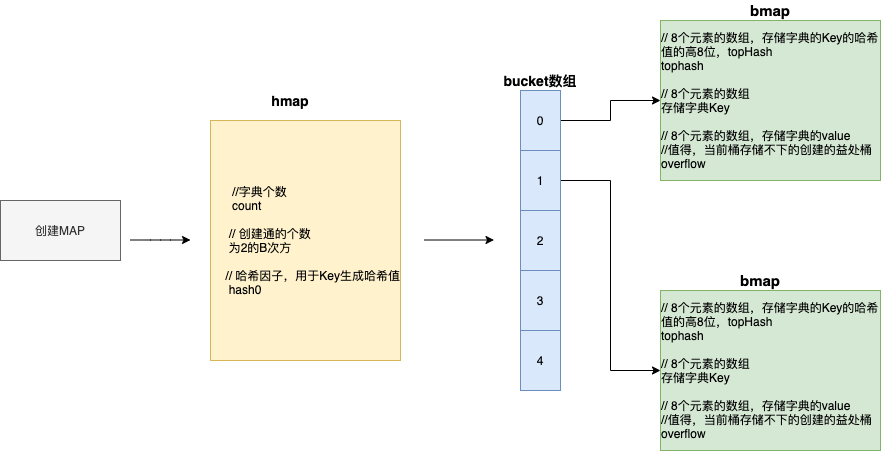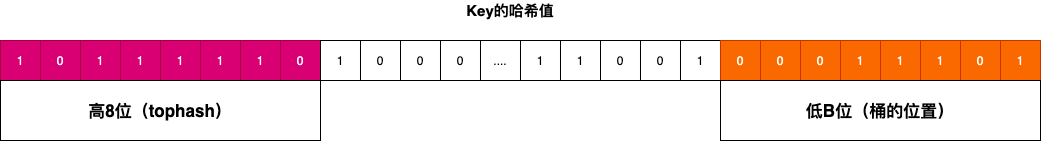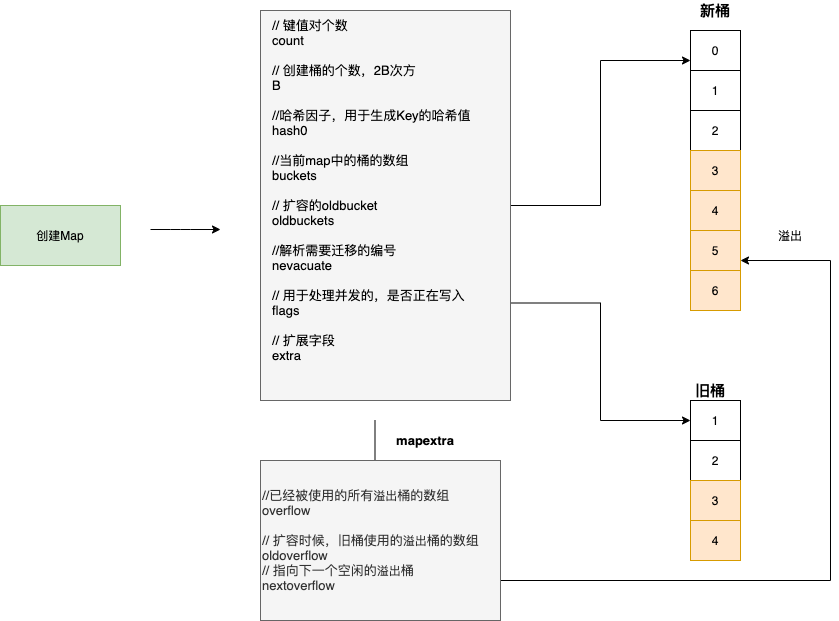# 深读 golang 中 map 后思考和借鉴ninetyhe# 简介

Golang 的 Map 概念不做过多解释，与 Python 的字典，Java 中的 Map 类似，都是 K、V 键值对的方式存储，其中键值是不可以重复，而且是无序的。Golang 中 Map 相对其他语言的一个特点就是：键值对支持 array 数组。

# 声明

mmap: =make([string]string)//make 初始化一个key是string，value是：stringmmap: =map[string]string{"key":"value"} // 普通初始化mmap:=new(map[string]string) // 这里初始化一个指针，但是需要赋值，不推荐var mmap map[string]string // 声明一个nil的map

# 常用操作

//获取map存储的键值对的个数length:=len(mmap)// 获取map容量 capacity:=cap(mmap) 无法执行会报错mmap["1"]="1" // 添加元素mmap["1"]="12" // 修改元素delete(mmap,"1")//删除for k,v:=range mmap {	// 遍历}// map 是否扩容，遍历引用都是指向的是同一块地址

# 底层剖析

Map 核心由 hmap 和 bmap 组成

### 结构类型

1. 最外面是 hmap 结构体，用 buckets 存放一些名字叫 bmap 的桶（数量不定，是 2 的指数倍）

2. bmap 是一种有 8 个格子的桶（一定只有 8 个格子），每个格子存放一对 key-value

3. bmap 有一个 overflow，用于连接下一个 bmap（溢出桶）

4. hmap 还有 oldbuckets，用于存放老数据（用于扩容时）

5. mapextra 用于存放非指针数据（用于优化存储和访问），内部的 overflow 和 oldoverflow 实际还是 bmap 的数组。

#### 对应源码

type hmap struct {	// Note: the format of the hmap is also encoded in cmd/compile/internal/gc/reflect.go.	// Make sure this stays in sync with the compiler's definition.	count     int // # live cells == size of map.  Must be first (used by len() builtin)	flags     uint8	B         uint8  // log_2 of # of buckets (can hold up to loadFactor * 2^B items)	noverflow uint16 // approximate number of overflow buckets; see incrnoverflow for details	hash0     uint32 // hash seed	buckets    unsafe.Pointer // array of 2^B Buckets. may be nil if count==0.	oldbuckets unsafe.Pointer // previous bucket array of half the size, non-nil only when growing	nevacuate  uintptr        // progress counter for evacuation (buckets less than this have been evacuated)	extra *mapextra // optional fields}### 初始化

1. 创建一个 hmap 结构体对象

2. 上传一个哈希因子 hash0 并赋值给 hmap 对象中，由于后期给 key 创建哈希值

3. 根据 hint=10，根据算法规则来创建 B

4. 根据 B 创建桶，并存放在 buckets 数组中：

• 当 B<4 的时候，创建桶的个数规则为：2^B 次方；

• 当 B>=4 的时候，创建桶的数量是：2^B+2^(B-4)（标准桶+益处桶）#### 对应源码

func makemap(t *maptype, hint int, h *hmap) *hmap {	mem, overflow := math.MulUintptr(uintptr(hint), t.bucket.size)	if overflow || mem > maxAlloc {		hint = 0	}	// initialize Hmap	if h == nil {		h = new(hmap)	}	h.hash0 = fastrand()	// Find the size parameter B which will hold the requested # of elements.	// For hint < 0 overLoadFactor returns false since hint < bucketCnt.	B := uint8(0)	for overLoadFactor(hint, B) {		B++	}	h.B = B	// allocate initial hash table	// if B == 0, the buckets field is allocated lazily later (in mapassign)	// If hint is large zeroing this memory could take a while.	if h.B != 0 {		var nextOverflow *bmap		h.buckets, nextOverflow = makeBucketArray(t, h.B, nil)		if nextOverflow != nil {			h.extra = new(mapextra)			h.extra.nextOverflow = nextOverflow		}	}	return h}

### 写入数据

1. 结合哈希因子与 key 生成一个哈希值（二进制）

2. 根据哈希值的后 B 位，并根据后 B 为的值来决定此键值对存放到哪个桶中（bmap）；（哈希值的高 8 位存储到 tophash 里面；）

3. 确定后写入数据，将 tophash，key，value 分部写入 bmap 的三个数组中，如果桶已经满，则通过 overflow 找到溢出桶，并在益处桶继续写入

4. hmap 中的个数 count++

#### 对应源码

// Like mapaccess, but allocates a slot for the key if it is not present in the map.func mapassign(t *maptype, h *hmap, key unsafe.Pointer) unsafe.Pointer {	if h == nil {		panic(plainError("assignment to entry in nil map"))	}	if raceenabled {		callerpc := getcallerpc()		pc := funcPC(mapassign)		racewritepc(unsafe.Pointer(h), callerpc, pc)		raceReadObjectPC(t.key, key, callerpc, pc)	}	if msanenabled {		msanread(key, t.key.size)	}	if h.flags&hashWriting != 0 {		throw("concurrent map writes")	}	hash := t.hasher(key, uintptr(h.hash0))	// Set hashWriting after calling t.hasher, since t.hasher may panic,	// in which case we have not actually done a write.	h.flags ^= hashWriting	if h.buckets == nil {		h.buckets = newobject(t.bucket) // newarray(t.bucket, 1)	}again:	bucket := hash & bucketMask(h.B)	if h.growing() {		growWork(t, h, bucket)	}	b := (*bmap)(unsafe.Pointer(uintptr(h.buckets) + bucket*uintptr(t.bucketsize)))	top := tophash(hash)	var inserti *uint8	var insertk unsafe.Pointer	var elem unsafe.Pointerbucketloop:	for {		for i := uintptr(0); i < bucketCnt; i++ {			if b.tophash[i] != top {				if isEmpty(b.tophash[i]) && inserti == nil {					inserti = &b.tophash[i]					insertk = add(unsafe.Pointer(b), dataOffset+i*uintptr(t.keysize))					elem = add(unsafe.Pointer(b), dataOffset+bucketCnt*uintptr(t.keysize)+i*uintptr(t.elemsize))				}				if b.tophash[i] == emptyRest {					break bucketloop				}				continue			}			k := add(unsafe.Pointer(b), dataOffset+i*uintptr(t.keysize))			if t.indirectkey() {				k = *((*unsafe.Pointer)(k))			}			if !t.key.equal(key, k) {				continue			}			// already have a mapping for key. Update it.			if t.needkeyupdate() {				typedmemmove(t.key, k, key)			}			elem = add(unsafe.Pointer(b), dataOffset+bucketCnt*uintptr(t.keysize)+i*uintptr(t.elemsize))			goto done		}		ovf := b.overflow(t)		if ovf == nil {			break		}		b = ovf	}	// Did not find mapping for key. Allocate new cell & add entry.	// If we hit the max load factor or we have too many overflow buckets,	// and we're not already in the middle of growing, start growing.	if !h.growing() && (overLoadFactor(h.count+1, h.B) || tooManyOverflowBuckets(h.noverflow, h.B)) {		hashGrow(t, h)		goto again // Growing the table invalidates everything, so try again	}	if inserti == nil {		// all current buckets are full, allocate a new one.		newb := h.newoverflow(t, b)		inserti = &newb.tophash		insertk = add(unsafe.Pointer(newb), dataOffset)		elem = add(insertk, bucketCnt*uintptr(t.keysize))	}	// store new key/elem at insert position	if t.indirectkey() {		kmem := newobject(t.key)		*(*unsafe.Pointer)(insertk) = kmem		insertk = kmem	}	if t.indirectelem() {		vmem := newobject(t.elem)		*(*unsafe.Pointer)(elem) = vmem	}	typedmemmove(t.key, insertk, key)	*inserti = top	h.count++done:	if h.flags&hashWriting == 0 {		throw("concurrent map writes")	}	h.flags &^= hashWriting	if t.indirectelem() {		elem = *((*unsafe.Pointer)(elem))	}	return elem}

### 读取数据

1. 结合哈希因子和 key 生成哈希值

2. 获取哈希值后 B 位来确定落在哪个桶（bmap）

3. 确定桶之后，再根据可以的哈希值的高 8 位来确定 tophash，最后根据 tophash 中和 key 查找的数据

4. 如果桶和溢出桶都不存在的时候，即数据不存在

#### 对应源码（由于 golang 会对应到具体类型的函数，这里以一个为例）

// mapaccess1 returns a pointer to h[key].  Never returns nil, instead// it will return a reference to the zero object for the elem type if// the key is not in the map.// NOTE: The returned pointer may keep the whole map live, so don't// hold onto it for very long.func mapaccess1(t *maptype, h *hmap, key unsafe.Pointer) unsafe.Pointer {	if raceenabled && h != nil {		callerpc := getcallerpc()		pc := funcPC(mapaccess1)		racereadpc(unsafe.Pointer(h), callerpc, pc)		raceReadObjectPC(t.key, key, callerpc, pc)	}	if msanenabled && h != nil {		msanread(key, t.key.size)	}	if h == nil || h.count == 0 {		if t.hashMightPanic() {			t.hasher(key, 0) // see issue 23734		}		return unsafe.Pointer(&zeroVal)	}	if h.flags&hashWriting != 0 {		throw("concurrent map read and map write")	}	hash := t.hasher(key, uintptr(h.hash0))	m := bucketMask(h.B)	b := (*bmap)(add(h.buckets, (hash&m)*uintptr(t.bucketsize)))	if c := h.oldbuckets; c != nil {		if !h.sameSizeGrow() {			// There used to be half as many buckets; mask down one more power of two.			m >>= 1		}		oldb := (*bmap)(add(c, (hash&m)*uintptr(t.bucketsize)))		if !evacuated(oldb) {			b = oldb		}	}	top := tophash(hash)bucketloop:	for ; b != nil; b = b.overflow(t) {		for i := uintptr(0); i < bucketCnt; i++ {			if b.tophash[i] != top {				if b.tophash[i] == emptyRest {					break bucketloop				}				continue			}			k := add(unsafe.Pointer(b), dataOffset+i*uintptr(t.keysize))			if t.indirectkey() {				k = *((*unsafe.Pointer)(k))			}			if t.key.equal(key, k) {				e := add(unsafe.Pointer(b), dataOffset+bucketCnt*uintptr(t.keysize)+i*uintptr(t.elemsize))				if t.indirectelem() {					e = *((*unsafe.Pointer)(e))				}				return e			}		}	}	return unsafe.Pointer(&zeroVal)}

### 扩容1. map 中的总数/桶个数 >6.5 ，引发翻倍扩容

2. 使用太多的益处桶

3. B <=15 ,引发等量扩容

4. B > 15 引发等量扩容

5. 扩容时候，新数据写入新桶

### 迁移

#### 对应源码

type mapextra struct {	// If both key and elem do not contain pointers and are inline, then we mark bucket	// type as containing no pointers. This avoids scanning such maps.	// However, bmap.overflow is a pointer. In order to keep overflow buckets	// alive, we store pointers to all overflow buckets in hmap.extra.overflow and hmap.extra.oldoverflow.	// overflow and oldoverflow are only used if key and elem do not contain pointers.	// overflow contains overflow buckets for hmap.buckets.	// oldoverflow contains overflow buckets for hmap.oldbuckets.	// The indirection allows to store a pointer to the slice in hiter.	overflow    *[]*bmap	oldoverflow *[]*bmap	// nextOverflow holds a pointer to a free overflow bucket.	nextOverflow *bmap}

### 思考

#### 结构设计

Golang 基本数据结构是：数组+数组+链表；由于在 bmap 中 golang 使用了高低位来快速定位到元素的位置，而不是像 Java 一样需要遍历链表，另外如果 golang 超过桶的个数后会通过指针指向下一个溢出桶，整体看是放弃了链表转红黑树的方式，这样在冲突过多的情况下，采用了使用空间换时间方式。

#### 扩容方式### ninetyhe

Technology Enthusiast 2019.08.20 加入

## 评论